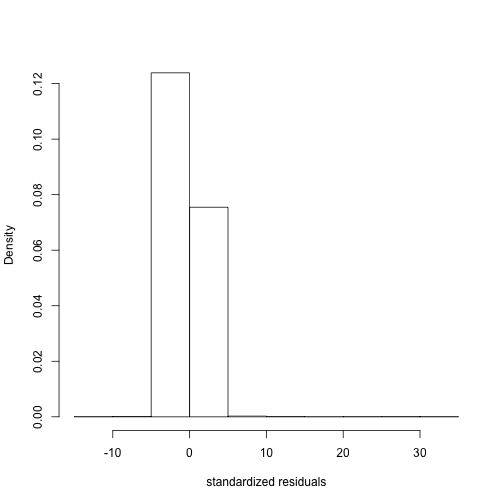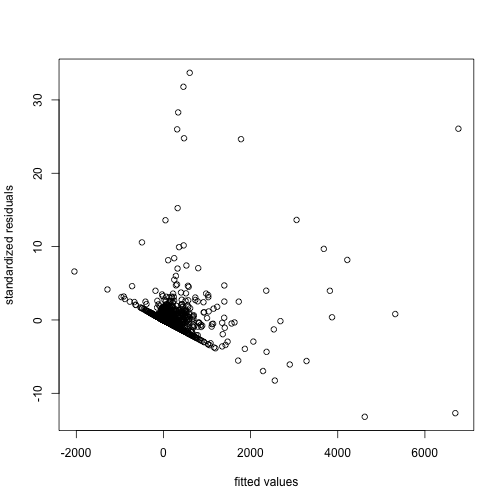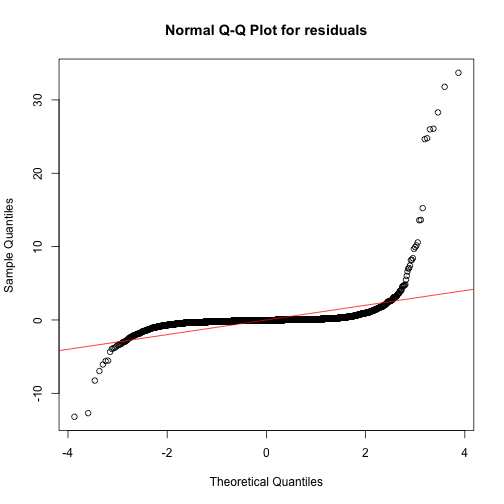# Abstract

The blin package provides methods for estimating “influence networks” from longitudinal bipartite relational data [@blin]. In this type of data, observations exist between disparate actor types, for example forum posts by users (see @zhou2007bipartite for many other examples). However, the size and number of posts by one user to a given forum may influence the the size and number of posts by another user to the same forum. This package focuses on inferring these influences between each pair of users in the data set. In this vignette we analyze a data set of forum posts at UC Irvine over 5 months in 2004 [@opsahl2013triadic]. We infer the influence networks in this data set (among both users and forums) using methods from @campbell2018 and @marrs2018.

# Introduction

We propose an autoregressive generarive model for longitudinal bipartite relational data. Any bipartite network at time $$t$$ may be represented by an $$S \times L$$ matrix $$Y_t$$, where, for example, $$S$$ is the number of users and $$L$$ is the number of forums. In our model, the current observation of the network $$Y_t$$ depends on the past observations $$Y_{-} := \{Y_r \}_{rlinmod} may be written as multiple linear regression with design matrix at time \(t$$ $$\mathbb{X}_B{(t)} = [(X{t}T \otimes IS) \ ; \ (I_L \otimes X{t}) ]. \label{eq_design}$$ Then, the complete design matrix is simply a column-wise stacking of each $$\mathbb{X}_B^{(t)}$$ for all $$t \in \{1,2,\ldots,T \}$$.

We note here that the influence networks in the BLIN model are not fully identifiable. For any $$c \in \mathbb{R}$$, the transformation $$\{ A, B\} \rightarrow \{A + c I_S, B - c I_L \}$$ gives the same mean for $$Y_t$$. The non-identifability means that we are unable to determine $$a_{ii}$$ and $$b_{jj}$$ separately, but that the sum $$a_{ii} + b_{jj}$$ is identifiable. When reporting results, we break out these sums of diagonals separately into an $$S \times L$$ matrix of $$\{a_{ii} + b_{jj} \}_{i,j}$$.

In this vignette, we analyze a data set of forum posts and generate the complete design matrix for the forum data. First, however, we briefly describe estimation procedures for the BLIN model.

### BLIN model estimation

As the BLIN model in \eqref{eq_linmod} may be written as a linear regression, least-squares estimation is one procedure for estimating the influence networks $$A$$ and $$B$$. This procedure is equivalent to the maximum likelihood estimator of \eqref{eq_linmod} under independent and homogeneous errors. To reduce memory demands, we use a block coordinate descent of the least squares criterion, $\{\hat{A}, \hat{B} \} = {\text argmin} \sum_t ||Y_t - A^T X_t - X_t B ||^2,$ to estimate $$A$$ and $$B$$. Block updates for $$\beta_i$$ are implemented as well when there are covariates in the model. Again, the linear regression setting means that the typical estimates of the standard errors of the entries in $$A$$ and $$B$$ are readily available.

When there are actors that are highly inlfuential, then we might expect reduced-rank structure in the networks $$A$$ and $$B$$. We use a block coordinate descent to estimate reduced rank networks $$A$$ and $$B$$. We do not provide standard errors for the reduced-rank estimators.

There may be settings where we expect very few influences. In these cases, the matrices $$A$$ and $$B$$ are sparse. We use the lasso-penalized regression available from \texttt{cv.glmnet(.)} to estimate entries in $$A$$ and $$B$$ [@friedman2010regularization]. We do not provide standard errors for this estimator as post-selection inference is an open research problem.

# Forum data analysis

We first analyze the data set from @opsahl2013triadic consisting of forum posts in a “Facebook-like online community”. These posts were made by 899 students to 552 forums over a period of six months in 2004 at the University of California at Irvine. We created a weighted bipartite relational dataset wherein each edge between a user and a forum in a given week consists of the number of characters the user posted to said forum in that particular week. For simplicity of analysis, we took only the 20 most active users and the 20 forums in which they were most active.

### Frquentist estimation

We first load the forum data set. We fit a separate intercept for each time period, contained in array $$Z$$ below.

data("forum")
Z <- array(0, c(dim(forum), dim(forum)))
for(i in 1:dim(forum)){  # fit a separate intercept for each week
Z[,,i,i] <- 1
}


We first fit the “full” BLIN model, that is the BLIN model without assuming reduced rank or sparse coefficient matrices. We also specify that the fitting should include the computation of standard errors with \texttt{calcses = TRUE}. For now, we fit with a lag of 1, that is the observation of the current bipartite network depends only on the previous observation. By default, the \texttt{summary(.)} method prints only the first ten entries in each estimated coefficient matrix $$A$$, $$B$$, and $$\beta$$.

fit1 <- blin_mle(forum, Z, lag=1, calcses=TRUE)    # full BLIN model
summary(fit1)   # summary

##
## Call:
## blin_mle(Y = forum, X = Z, lag = 1, calcses = TRUE)
##
##  A coefficients:
##         Estimate Std. Error t value Pr(|t| > 0)
##  [1,] -0.1833715  0.3175492 -0.5775      0.2818
##  [2,] -0.0352686  0.3226099 -0.1093      0.4565
##  [3,]  0.0104814  0.2953622  0.0355      0.4858
##  [4,] -0.0237206  0.2986102 -0.0794      0.4683
##  [5,] -0.0479666  0.2881523 -0.1665      0.4339
##  [6,]  0.0242739  0.4384547  0.0554      0.4779
##  [7,]  0.0112615  0.5156632  0.0218      0.4913
##  [8,]  0.0533002  0.3014539  0.1768      0.4298
##  [9,]  0.0156482  0.3281042  0.0477      0.4810
## [10,] -0.0033761  0.3353747 -0.0101      0.4960
##
##  ...
##  B coefficients:
##         Estimate Std. Error t value Pr(|t| > 0)
##  [1,]  0.8628059  0.1078017  8.0036   5.551e-16 ***
##  [2,] -0.0461510  0.2262656 -0.2040     0.41919
##  [3,]  0.0093909  0.0245076  0.3832     0.35079
##  [4,]  0.0024509  0.0827547  0.0296     0.48819
##  [5,]  0.0472683  0.0933560  0.5063     0.30632
##  [6,]  0.0787824  0.1353019  0.5823     0.28019
##  [7,] -0.0297080  0.0486200 -0.6110     0.27059
##  [8,]  0.1585432  0.3012536  0.5263     0.29935
##  [9,] -0.0206000  0.0131749 -1.5636     0.05896 .
## [10,] -0.0393621  0.1085479 -0.3626     0.35844
## ---
## Signif. codes:  0 '***' 0.001 '**' 0.01 '*' 0.05 '.' 0.1 ' ' 1
##
##  ...
##  beta coefficients:
##          Estimate  Std. Error t value Pr(|t| > 0)
##  [1,]  0.0000e+00  3.3897e-11  0.0000    0.500000
##  [2,]  6.6810e+01  1.9369e+01  3.4493    0.000281 ***
##  [3,]  5.9279e+01  2.3345e+01  2.5392    0.005555 **
##  [4,]  6.7325e+00  2.5731e+01  0.2616    0.396796
##  [5,]  3.5692e+01  2.0238e+01  1.7636    0.038901 *
##  [6,] -1.1437e+01  1.9127e+01 -0.5980    0.274925
##  [7,]  1.2126e+01  1.6319e+01  0.7430    0.228727
##  [8,]  1.0986e+01  1.6996e+01  0.6464    0.259016
##  [9,]  2.3475e+01  1.6644e+01  1.4104    0.079215 .
## [10,]  1.8060e+01  1.6483e+01  1.0956    0.136618
## ---
## Signif. codes:  0 '***' 0.001 '**' 0.01 '*' 0.05 '.' 0.1 ' ' 1
##
##  ...
##  diagAB coefficients:
##         Estimate Std. Error t value Pr(|t| > 0)
##  [1,]  0.6794344  0.3427258  1.9824    0.023715 *
##  [2,] -0.2317918  0.1108380 -2.0913    0.018252 *
##  [3,]  0.1735847  0.2044650  0.8490    0.197949
##  [4,] -0.0026498  0.1746987 -0.0152    0.493949
##  [5,]  0.5115485  0.2161590  2.3665    0.008978 **
##  [6,]  0.4531423  0.3737440  1.2124    0.112672
##  [7,]  0.1820491  0.1049059  1.7354    0.041339 *
##  [8,]  0.1157518  0.3201479  0.3616    0.358841
##  [9,]  0.1913520  0.1890374  1.0122    0.155711
## [10,]  0.1027274  0.1478091  0.6950    0.243527
## ---
## Signif. codes:  0 '***' 0.001 '**' 0.01 '*' 0.05 '.' 0.1 ' ' 1
##
##  ...

plot(fit1)To demonstrate the remaining frequentist estimation procedures of the BLIN model, we fit the full, reduced rank, and sparse BLIN models to the forum data set. We do so for a range of lags and print out the in-sample $$R^2$$ values. The results suggest that a lag of $$1$$ may be sufficient.

lags <- 1:2
R2 <- matrix(0, length(lags), 3)  ;  colnames(R2) <- c("full", "reduced_rank", "sparse")
for(i in 1:length(lags)){
fit <- blin_mle(forum, Z, lag=lags[i])
R2[i,1] <- fit$R2 fit <- blin_mle(forum, Z, lag=lags[i], rankA=5, rankB=5, type="reduced_rank") R2[i,2] <- fit$R2
fit <- blin_mle(forum, Z, lag=lags[i], type="sparse")
R2[i,3] <- fit\$R2
}
print(R2)

##           full reduced_rank    sparse
## [1,] 0.3335198    0.3179516 0.1160826
## [2,] 0.3289827    0.3164759 0.1186958


The residual plots following the least-squares estimates of the BLIN model do not appear normally distributed. It may be appropriate to cast the BLIN model as a generalized linear model with an appropriate link. We provide a function to build the design matrix based on \eqref{eq_design}. This design matrix may be large, as it is of dimension $$SLT \times (S^2 + L^2 + p)$$. We leave further investigation of this data set to the reader.
Xreg <- build_design(forum, X=Z, lag=1)   # design matrix for lag=1

##  9200  824